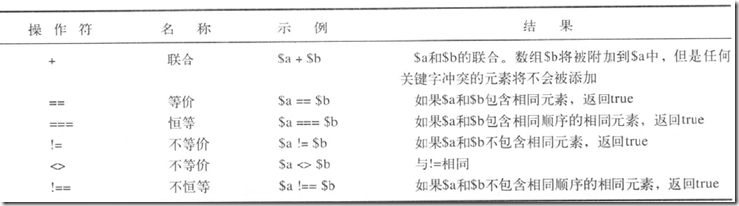1. 数字索引数组；
2. 非数字索引数组；
3. 数组操作符；
4. 二维数组和多维数组；
5. 数组排序；
6. 多维数组排序；
7. 对数组进行重排序；
8. 执行其他数组操作；

# 数字索引数组

### 新建数组：

$phones = array('htc','moto','nokia'); 或： $phones = 'htc';
$phones = 'moto';$phones = 'nokia';

echo $phones.'--'.$phones;

$number = range(1,10); 此函数还有一个可选的第三个参数，这个参数允许设定值之间的步幅，如，创建一个从1到10的奇数， $number = range(1,10,2);

range()函数也可以对字符进行操作，如，需要一个从a到z的字符列表，就可以以以下操作

$number = range('a','z'); ### 访问数组： 1.for循环 $phones = array('htc','moto','nokia');
for($i=0;$i<3;$i++){ echo "$phones[$i]<br />"; } 2.foreach（专门为数组而设计） $phones = array('htc','moto','nokia');
foreach($phones as$v){
echo "$v<br />"; } # 非数字索引数组（相关数组） 在$phones数组中，允许PHP指定一个默认的索引。也就意味着所添加的第一个元素的索引为0，第二个为1等等。PHP还支持相关数组（非数字索引数组），以下统称为相关数组，可以将每个变量的值与 任何关键字或索引关联起来。类似于C#中Dictionary<TKey,TValue>。

$phones = array('htcG6' => 2400,'motoI9' => 2500,'nokiaN8' => 2890); 已上单引号中的是关键字，=>后边的是值。 ### 访问数组： 因为相关数组中的索引不是数字，所以就不能用for语句进行操作了，但是可以使用foreach循环或list()和each()结构。 1.foreach访问 foreach($phones as $key =>$value){
echo "$key:$value<br />";
}

2.each访问

while($element = each($phones)){
echo $element['key']; //$element
echo ':';
echo $element['value']; //$element
echo '<br />';
}

each()函数，将返回数组当前元素，并将下一个元素作为当前元素，在while循环中，将按顺序输出。$element也是一个数组。当条用each()时，用位置key或0包含当前元素的关键字，而位置value或1包含当前元素的值。 3.list访问 while(list($name,$price) = each($phones)){
echo $name; echo ':'; echo$price;
echo '<br />';
}

list()函数和方法2中$element变量的使用方法类似，但是list能够重新指定自定义变量，指向数组用一组元素的关键字和值。这个比方法2更方便，明了。\ # 数组操作符 数组间的操作：# 二维数组和多维数组 二维数组，以及多维数组和一维数组的建立和循环类似，只是在一维的基础上，在加数组。 $phones = array(array('xiaomi','小米手机',1900),
array('htc','G6',2400),
array('nokia','n8',3200));

## 方法一，直接使用数字索引

echo '|'.$phones.'|'.$phones.'|'.$phones.'<br />'; echo '|'.$phones.'|'.$phones.'|'.$phones.'<br />';
echo '|'.$phones.'|'.$phones.'|'.$phones.'<br />'; ## 方法二，使用双重for循环 for($row=0;$row<3;$row++){
for($col=0;$col<3;$col++){ echo '|'.$phones[$row][$col];
}
echo '<br />';
}

$phones = array(array('name'=>'xiaomi','desc'=>'小米手机','price'=>1900), array('name'=>'htc','desc'=>'g6','price'=>2400), array('name'=>'nokia','desc'=>'n8','price'=>3200)); 要遍历已上的数据，可以使用如下方法。 ## 方法一 for($row=0;$row<3;$row++){
echo '|'.$phones[$row]['name'].'|'.$phones[$row]['desc'].'|'.$phones[$row]['price'].'<br />';
}

## 方法二

for($row=0;$row<3;$row++){ while(list($key,$value) = each($phones[$row])){ echo "|$value";
}
echo '<br />';
}



# 数组排序

## 1，sort()函数

如下代码，将按字符顺序排序

     $products = array('htc','nokia','moto'); foreach($products as $value){ echo "$value<br />";
}
sort($products); echo '------------<br />'; foreach($products as $value){ echo "$value<br />";
}

也可以按数字的升序进行排序

     $products = array(2,100,5); foreach($products as $value){ echo "$value<br />";
}
sort($products); echo '------------<br />'; foreach($products as $value){ echo "$value<br />";
}

输出2,5,100

该函数的第二个参数是可选的。可以传递SORT_GEGULAR(默认值),SORT_NUMERIC或SORT_STRINT。指定排序类型的功能是非常有用的，例如，当

比较可能包含有数字2和12的字符串时，从数字的角度看2小于12，但从字符的角度看，‘12’确小于‘2’

注意：sort()函数时区分大小写的。

## 2，asort()函数和ksort()函数

如果对相关数组中的Key或Value在排序时一致，就需要使用这两个函数了。

ksort函数：针对相关数组中的Key进行升序排序

     $phones = array('htcG6' => 2400,'nokiaN8' => 2890,'motoI9' => 3200); ksort($phones);

输出：htcG6,motoI9,nokiaN8

asort函数：针对相关数组中的Value进行升序排序

     $phones = array('htcG6' => 2400,'nokiaN8' => 2890,'motoI9' => 3200); asort($phones);

输出：2400,2890,3200

## 3，反向排序

上面说的都是按照升序进行排序，如果需要降序，则要使用每一个函数所对应的降序函数。

sort() ==>rsort()

ksort() ==>krsort()

asort() ==>arsort()

使用方法都是一样的。

# 多维数组排序

## 1，用户自定排序

$phones = array(array('name'=>'xiaomi','desc'=>'小米手机','price'=>1900), array('name'=>'htc','desc'=>'g6','price'=>1400), array('name'=>'nokia','desc'=>'n8','price'=>3200)); 如果对以上的二维相关数据进行排序，就比较麻烦了。因为包含三种条件，不同的条件，排序算法可能都不一样，所以需要用户自定编写排序算法。 usort()函数，提供二个参数，第一个参数，是需要排序的对象，第二个参数是自定义函数。如下： $phones = array(array('name'=>'xiaomi','desc'=>'小米手机','price'=>1900),
array('name'=>'htc','desc'=>'g6','price'=>1400),
array('name'=>'nokia','desc'=>'n8','price'=>3200));
function compare($x,$y){
	if($x['price'] ==$y['price']){
return 0;
}else	if($x['price'] <$y['price']){
		return -1;
}else{
return 1;
}
}

usort($phones,'compare');  for($row=0;$row<3;$row++){
while(list($key,$value) = each($phones[$row])){
echo "|$value"; } echo '<br />'; } 上面的compare()函数接受两个需比较的数组。最后按照价格升序输出。 如果需要对name进行排序的话，只需把compare()函数中的price替换成name即可。 usort()中的”u”代表”user”，因为这个函数要求传入用户自定义函数。asort和ksort对应的版本uasort和uksort也要求传入用户自定义函数。 类似于asort和ksort，当对相关数组进行排序时，uasort和uksort才会被使用。 ## 2，反向用户排序 函数sort(),asort()，ksort()都分别对应一个带字母“r”的反向排序函数。用户定义的排序没有反向体。但只要把用户自定义函数的返回值取反即可。如： function compare($x,$y){ if($x['price'] == $y['price']){ return 0; }else if($x['price'] > $y['price'] ){ return -1; }else{ return 1; } } # 对数组进行重新排序 ## 1，使用shuffle()函数 我们可能在需要在10个广告中随机显示3个广告，那么这个函数就派上用场了。如下： $myChars = range('a','z');
shuffle($myChars); for($i=0;$i<3;$i++){
echo $myChars[$i].'<br />';
}

$myChars = range(1,10);$rChars = array_rand($myChars,2); //第一参数为数组对象，第二个参数可选，默认1。返回多少个随机的元素。 echo$rChars.'-'.$rChars; ## 2，使用array_reverse()函数 如果对于一个数组，我们想把它翻转，那么这个函数就有用武之地了。比如：1,2,3,4，我们想输出4,3,2,1，虽然for循环可以，但是需要些三行代码。 array_reverse()函数使用如下： $numbers = range(1,10);
$numbers = array_reverse($numbers);
foreach($numbers as$value){
echo $value.'<br />'; } # 执行其他的数组操作 ## 1，在数组中浏览：each()，current()，reset()，end()，next()，pos()和prev() each()间接的使用了数组的指针，其他的函数都是直接使用操作这个指针。 如果创建一个新数组，那么当前指针就被初始化，并指向第一个元素，调用current($array_name)将返回第一个元素。

prev()函数正好和next()相反。

## 2，对数组的每一个元素应用任何函数：array_walk()

array_walk()函数的原型如下：

bool array_walk(array arr,string func,[mixed userdata]);

$numbers = range(1,10); function show_even_number($value){
if($value % 2 ==0){ echo$value.'<br />';
}
}
array_walk($numbers,'show_even_number'); 上面的代码，只显示偶数。 我们也可以对这个数组中的每一个元素进行修改等等之类的操作。如下： $numbers = range(1,10);
function replace_number(&$value,$key,$userdata){$value = $value *$userdata;
}
array_walk($numbers,'replace_number',2); foreach($numbers as $value){ echo$value .'<br />';
}

echo count($numbers); //sizeof($numbers)

array_count_values()函数，返回数组中每一个元素，出现的频率数的一个相关数组。如下：

$numbers = array(5,1,8,5,3,0,4,3,5);$ac = array_count_values($numbers); while(list($key,$value) = each($ac)){
echo $key.'--'.$value.'<br />';
}

5--3
1--1
8--1
3--2
0--1
4--1

## 4，将数组转换成标量变量：extract()

extract()函数的作用是将一个数组转换成一系列的标量变量，这些变量的名称必须是数组中的Key，而变量值则是数组中的值。如下：

$phones = array('name' => 'xiaomi','price'=>1999,'os' => 'android'); extract($phones);
echo "$name =>$price => \$os";

posted @ 2011-09-06 16:03  兴百放  阅读(...)  评论(...编辑  收藏